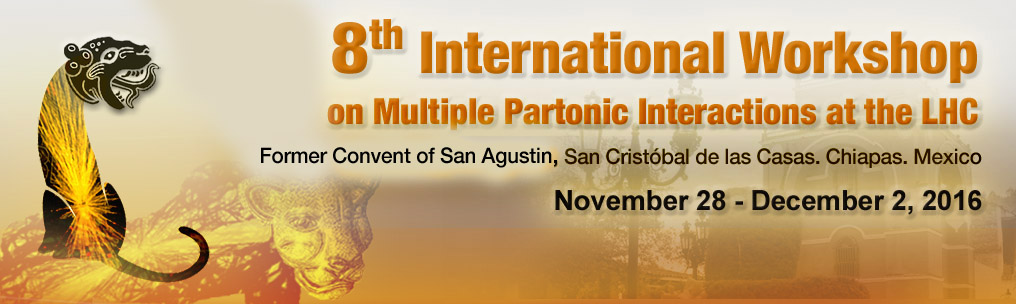#8th International Workshop on Multiple Partonic Interactions at the LHC

from 28 November 2016 to 2 December 2016
Hotel Rincón del Arco
Mexico/General timezone
Home > Timetable > Session details > Contribution details

# Contribution

Hotel Rincón del Arco - Salón Giralda

# Angular correlations as a function of multiplicity in pp and p-Pb collisions in the ATLAS experiment

## Speakers

• Dr. Deepak KAR

## Abstract content

ATLAS measurements of correlations between particle pairs in relative azimuthal angle ($\Delta\phi$) and pseudorapidity separation ($\Delta\eta$), in $pp$ collisions at $\sqrt{s}$=2.76, 5.02 and 13~TeV, and in $p$+Pb collisions at $\sqrt{s_{\mathrm{NN}}}$=5.02~TeV are presented. Prior measurements have shown that in $pp$ collisions with a large multiplicity of produced particles, a long-range structure,commonly called the ridge'', develops along $\Delta\eta$ at $\Delta\phi\sim$0. However, due to the presence of the large away-side jet, the full $\Delta\phi$ dependence of the long-range correlation could not be studied previously. In this analysis, a template fitting procedure is implemented to determine the contributions from dijets to the correlations, using low-multiplicity events, and extract the genuine long-range correlation. The long-range correlations are shown to be present even in events with a small multiplicity of produced particles, implying that the long-range correlations are not unique to rare high multiplicity events. The properties of the correlation are shown to be remarkably similar to that observed in $p$+Pb collisions. Study of these long range correlations using multi-particle cumulants are also presented. New results on how the long-range correlations are affected by the presence or absence of hard processes in the $pp$ collision are presented.